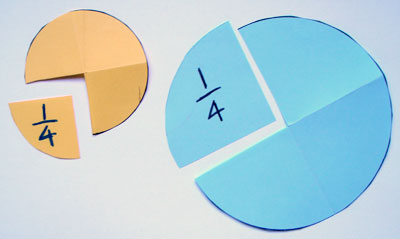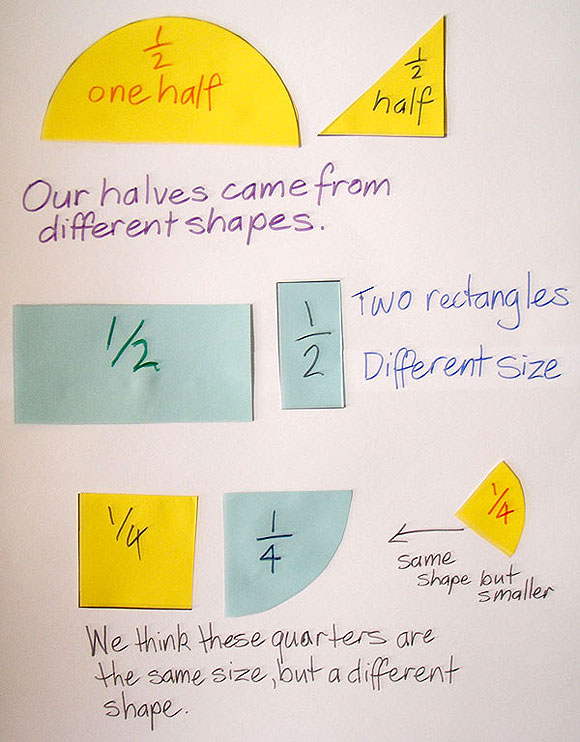# Cut and find

This activity focuses on halves and quarters but any fractions can be used.

Pose the question:

When is one-half larger than one-quarter?

and discuss possibilities as a class.

Prepare multiple copies of four paper shapes: a large and small circle, and a large and small square. The template of different shapes might be helpful.

Each student takes one of the four different paper shapes and:

• folds the shape to make a fraction specified by the teacher
• labels one of the equal parts with the appropriate unit fraction
• cuts the labelled piece away from the whole.

Ensure that all possibilities are covered, i.e. small circles divided into halves, small circles divided into quarters, large circles divided into halves, large circles divided into quarters, small squares divided into halves, small squares divided into quarters, large squares divided into halves and large squares divided into quarters.Quarters cut from different sized circles.

Students form groups and find someone with a labelled fraction piece that is:

• the same unit fraction
Is the other piece the same or different? Why?
• a larger fraction
How do you know that it is larger?
• a smaller piece of paper
Is the fraction the same or different? Why?
• a different shaped piece
Is the fraction the same or different? Why?

Each group prepares a poster to report several conclusions and explanations from their comparisons.Sample poster.